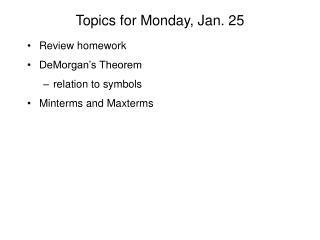# Topics for Monday, Jan. 25 - PowerPoint PPT PresentationDownload PresentationTopics for Monday, Jan. 25

Topics for Monday, Jan. 25
Download Presentation## Topics for Monday, Jan. 25

- - - - - - - - - - - - - - - - - - - - - - - - - - - E N D - - - - - - - - - - - - - - - - - - - - - - - - - - -
##### Presentation Transcript

1. Topics for Monday, Jan. 25 • Review homework • DeMorgan’s Theorem • relation to symbols • Minterms and Maxterms

2. prove x(x + y) = x • 1. (10 points)Hint: Use duality and the proof already shown • From Friday, x+xy = x 1 + xy = x(1+y) = x 1 = x • Apply duality   , 1  0 x(x+y) x(x+y)=(x+0)(x+y) = x+(0  y) = x+0 = x

3. Sum-of-Products form 2. (10 points) g = (a + c)(d + ef) Let x=(a+c), y=d, z=ef and x(y+z) = xy+xz, so (a+c)(d+ef) =(a+c)d + (a+c)ef = ad + cd + aef + cef

4. Product-of-Sums form 3. (10 points) h=abc + d + e Let (d+e)=x, ab = y, c = z and x+yz = (x+y)(x+z), so abc + d + e = (ab + d + e)(c + d + e) Let (d+e) = x and ab + d + e looks like x + yz, so abc + d + e = (a + d + e)(b + d + e)(c + d + e)

5. DeMorgan’s Theorem • DeMorgan’s Theorem provides the final link in taking any expression, including an expression with NAND or NOR, and converting it to S-o-P or P-o-S form. • (x+y)’ = x’ • y’ (x • y)’ = x’ + y’

6. DeMorgan and Symbols (x+y)’ = x’ • y’ f= (x+y)’= x  y What symbol would express f = x’ • y’? A logic mismatch between the symbol and the logic convention introduces the NOT operation

7. NAND, NOR equivalents (x+y)’ = x’ • y’ (x • y)’ = x’ + y’

8. Minterm • A minterm is composed of every input variable (or its complement) ANDed together • The truth table for a minterm in an n-variable system has one “1” and 2n - 1 ”0”’s • A minterm is denoted by a lowercase m and the (equivalent) number of the row on which the “1” occurs • a’b’c’ has a “1” for inputs 000 and is called minterm 0 and denoted m0 • ab’c has a”1” for inputs 101 and is called minterm 5 and denoted m5

9. Truth Tables to Functions • Minterms provide a way to write the function for any truth table. • The minterms for every row where a “1” occurs are OR’ed together. ( x + 1 = 1)

10. a’b’c’ 1 0 0 0 0 0 0 0 a’bc’ 0 0 1 0 0 0 0 0 ab’c 0 0 0 0 0 1 0 0 abc’ 0 0 0 0 0 0 1 0 a’b’c’ + a’bc’ + ab’c + abc’ Write a function abc f 000 1 001 0 010 1 011 0 100 0 101 1 110 1 111 0 f =

11. Maxterm • A Maxterm is composed of every input variable (or its complement) ORed together • The truth table for a Maxterm in an n-variable system has one “0” and 2n - 1 ”1”’s • A Maxterm is denoted by a uppercase M and the (equivalent) number of the row on which the “0” occurs • a’+b’+c’ has a “0” for inputs 111 and is called Maxterm 7 and denoted M7 • a+b’+c has a”0” for inputs 010 and is called Maxterm 2 and denoted M2

12. Truth Tables to Functions • Maxterms provide a way to write the function for any truth table. • The Maxterms for every row where a “0” occurs are AND’ed together. ( x • 0 = 0)

13. (a+b+c’) 1 0 1 1 1 1 1 1 (a+b’+c’) 1 1 1 0 1 1 1 1 (a’+b+c) 1 1 1 1 0 1 1 1 (a’+b’+c’) 1 1 1 1 1 1 1 0 (a+b+c’) (a+b’+c’) (a’+b+c) (a’+b’+c’) Write a function (Maxterms) abc f 000 1 001 0 010 1 011 0 100 0 101 1 110 1 111 0 f =

14. Canonical Forms and Lists • The canonical minterm and Maxterm forms list every literal and operator • f = a’b’c’ + a’bc’ + ab’c + abc’ • f= (a+b+c’)(a+b’+c’)(a’+b+c)(a’+b’+c’) • A list is a shorthand method of displaying which rows the 0’s and 1’s occupy • f = m(0,2,5,6) = M(1,3,4,7)

15. Homework for Wednesday, Jan. 27 • Problem 1. (10 points) • a) Write the electrical truth table for the gate symbol shown below • b) Write the operation for the gate symbol shown below when • Input=P.A. and Output=N.A • Input=P.A. and Output=P.A. • Input=N.A. and Output=N.A. • Input=N.A. and Output=P.A.

16. abcd f 0000 0001 0010 0011 0100 0101 0110 0111 1000 1001 1010 1011 1100 1101 1110 1111 1 0 1 0 111100000101 Problem 2. (20 pts) a)Write the minterm expression for the function f(canonical form and list) b) Write the Maxterm expression for the function f (canonical form and list)